Selenium

Selenium, Recone Kits

Selenium Replacement Diaphragm for DH200HM and DH200E EUR 18,00excl. VAT: € 15.13 / \$ 16.94
Selenium Replacement Diaphragm for ST200 EUR 9,40excl. VAT: € 7.90 / \$ 8.85
Selenium Replacement Diaphragm for D2500TI-ND,D220TI, D220TI-OMF, 8 Ohm EUR 18,80excl. VAT: € 15.80 / \$ 17.69
Selenium Replacement Diaphragm for D405 EUR 38,00excl. VAT: € 31.93 / \$ 35.76
Selenium Replacement Diaphragm for ST300-SLF, ST320 EUR 11,40excl. VAT: € 9.58 / \$ 10.73
Selenium Replacement Diaphragma for ST400 EUR 19,00excl. VAT: € 15.97 / \$ 17.88
Selenium ADF25-25, Adaptor, 3 Hole Bolt on to 25mm- not available

Compression driver for Horns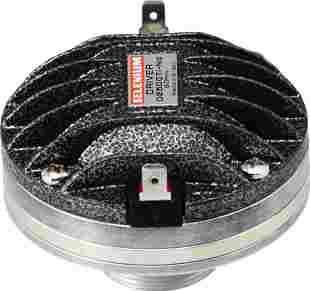open a bigger photo

D2500Ti-Nd 8 Ohm       order no. sel-28031043       EUR 117,00excl. VAT: € 98.32 / \$ 110.12
1 inch high frequency compression driver.
• power handling (continuous/programme) = 120/160 W
• frequency range = 1000-22000 Hz
• impedance R = 8 Ohm
• sound pressure level SPL = 111 dB (2,83V; 1m)
• DC resistance Re = 6 Ohm
• voice coil diameter = 44 mm
• overall diameter d = 80 mm
• mounting depth (not countersunk) t = 56,4 mm
• mass m = 0,73 kg
PDF data sheetopen a bigger photo

D3505Ti-Nd DPD       order no. sel-28031046       EUR 394,00excl. VAT: € 331.09 / \$ 370.82
2 inch high frequency compression driver.
• power handling (continuous/programme) = 150 W
• frequency range = 500-20000 Hz
• impedance R = 16 Ohm
• sound pressure level SPL = 111 dB (2,83V; 1m)
• DC resistance Re = 8,5 Ohm
• voice coil diameter = 75 mm
• overall diameter d = 126 mm
• mounting depth (not countersunk) t = 71 mm
• mass m = 2,8 kg
PDF data sheetopen a bigger photo

D3501Ti-Nd DPD       order no. sel-28031060- not available
2 inch high frequency compression driver.
• power handling (continuous/programme) = 150 W
• frequency range = 500-25000 Hz
• impedance R = 8 Ohm
• sound pressure level SPL = 111 dB (2,83V; 1m)
• DC resistance Re = 4,8 Ohm
• voice coil diameter = 75 mm
• overall diameter d = 126 mm
• mounting depth (not countersunk) t = 109 mm
• mass m = 3,1 kg
PDF data sheetopen a bigger photo

D3300Ti DPD       order no. sel-28031021- not available
2 inch high frequency compression driver.
• power handling (continuous/programme) = 150 W
• frequency range = 500-25000 Hz
• impedance R = 8 Ohm
• sound pressure level SPL = 110 dB (2,83V; 1m)
• DC resistance Re = 4,8 Ohm
• voice coil diameter = 75 mm
• overall diameter d = 176 mm
• mounting depth (not countersunk) t = 97 mm
• mass m = 5,63 kg
PDF data sheetopen a bigger photo

D305       order no. sel-28031005       EUR 153,00excl. VAT: € 128.57 / \$ 144.00
2 inch high frequency compression driver.
• power handling (continuous/programme) = 100/150 W
• frequency range = 400-9000 Hz
• impedance R = 8 Ohm
• sound pressure level SPL = 110 dB (2,83V; 1m)
• DC resistance Re = 6 Ohm
• voice coil diameter = 75 mm
• overall diameter d = 169 mm
• mounting depth (not countersunk) t = 76 mm
• mass m = 5 kg
PDF data sheetopen a bigger photo

D405       order no. sel-28031006       EUR 238,00excl. VAT: € 200.00 / \$ 224.00
2 inch high frequency compression driver.
• power handling (continuous/programme) = 150/200 W
• frequency range = 300-7000 Hz
• impedance R = 8 Ohm
• sound pressure level SPL = 110 dB (2,83V; 1m)
• DC resistance Re = 6 Ohm
• voice coil diameter = 100 mm
• overall diameter d = 205 mm
• mounting depth (not countersunk) t = 86 mm
• mass m = 8,3 kg
PDF data sheetopen a bigger photo

D405Ti       order no. sel-28031011- not available
2 inch high frequency compression driver.
• power handling (continuous/programme) = 150/200 W
• frequency range = 300-7000 Hz
• impedance R = 8 Ohm
• sound pressure level SPL = 110 dB (2,83V; 1m)
• DC resistance Re = 6 Ohm
• voice coil diameter = 100 mm
• overall diameter d = 205 mm
• mounting depth (not countersunk) t = 86 mm
• mass m = 8,3 kg
PDF data sheetopen a bigger photo

D4400Ti       order no. sel-28031016       EUR 399,00excl. VAT: € 335.29 / \$ 375.53
2 inch high frequency compression driver.
• power handling (continuous/programme) = 200/250 W
• frequency range = 400-20000 Hz
• impedance R = 8 Ohm
• sound pressure level SPL = 112 dB (2,83V; 1m)
• DC resistance Re = 4,8 Ohm
• voice coil diameter = 100 mm
• overall diameter d = 230 mm
• mounting depth (not countersunk) t = 163 mm
• mass m = 10,2 kg
PDF data sheet

Hornopen a bigger photo

HC23-25       order no. sel-28056014       EUR 15,40excl. VAT: € 12.94 / \$ 14.49
Horn for a compression driver with 1 inch throat.
• nominal angle (HxV) = 100x40 Deg
• Cut off frequency : 600 Hz
• over all dimensions (HxWxD) = 128x254x145 mm
• net weight = 0,34 kg
• material: high quality
PDF data sheetopen a bigger photo

HM11-25       order no. sel-28056033       EUR 9,40excl. VAT: € 7.90 / \$ 8.85
Horn for a compression driver with 1 inch throat.
• nominal angle (HxV) = 60x60 Deg
• Cut off frequency : 1200 Hz
• over all dimensions (HxWxD) = 115x115x75 mm
• net weight = 0,1 kg
• material: high quality
PDF data sheetopen a bigger photo

HL14-25       order no. sel-28056001- not available
Horn for a compression driver with 1 inch throat.
• nominal angle (HxV) = 45x45 Deg
• Cut off frequency : 600 Hz
• over all dimensions (HxWxD) = 156x156x258 mm
• net weight = 0,21 kg
• material: high quality
PDF data sheet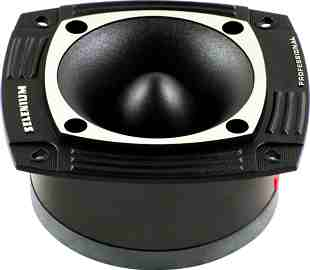open a bigger photo

ST304       order no. sel-28003015       EUR 39,00excl. VAT: € 32.77 / \$ 36.71
46 mm tweeter with high quality cone.
• power handling (continuous/programme) = 40/80 W
• frequency range = 3500-18000 Hz
• impedance R = 8 Ohm
• sound pressure level SPL = 106 dB (2,83V; 1m)
• DC resistance Re = 6 Ohm
• voice coil diameter = 46 mm
• mounting diameter d = 104 mm
• overall diameter d = 124 mm
• mounting depth (not countersunk) t = 63 mm
• mass m = 1,1 kg
PDF data sheetopen a bigger photo

ST322       order no. sel-ST322       - not available (_)
46 mm tweeter with high quality cone.
• power handling (continuous/programme) = 50/100 W
• frequency range = 3500-20000 Hz
• impedance R = 8 Ohm
• sound pressure level SPL = 105 dB (2,83V; 1m)
• DC resistance Re = 6,1 Ohm
• voice coil diameter = 46 mm
• mounting diameter d = 107 mm
• overall diameter d = 124 mm
• mounting depth (not countersunk) t = 90 mm
• mass m = 1,5 kg
PDF data sheetopen a bigger photo

DT150       order no. sel-28058002       EUR 43,27excl. VAT: € 36.36 / \$ 40.72
1 inch high frequency compression driver.
• power handling (continuous/programme) = 80/150 W
• frequency range = 1500-15000 Hz
• impedance R = 8 Ohm
• sound pressure level SPL = 108 dB (2,83V; 1m)
• DC resistance Re = 8 Ohm
• voice coil diameter = 25 mm
• overall diameter d = 115 mm
• mounting depth (not countersunk) t = 120 mm
• mass m = 0,8 kg
PDF data sheetopen a bigger photo

ST350       order no. sel-28003017       EUR 58,00excl. VAT: € 48.74 / \$ 54.59
42 mm tweeter with high quality cone.
• power handling (continuous/programme) = 150/200 W
• frequency range = 2500-20000 Hz
• impedance R = 8 Ohm
• sound pressure level SPL = 111 dB (2,83V; 1m)
• DC resistance Re = 6,2 Ohm
• voice coil diameter = 42 mm
• mounting diameter d = 104 mm
• overall diameter d = 130 mm
• mounting depth (not countersunk) t = 98 mm
• mass m = 1,42 kg
PDF data sheetopen a bigger photo

ST400       order no. sel-75003029       EUR 81,00excl. VAT: € 68.07 / \$ 76.24
42 mm tweeter with high quality cone.
• power handling (continuous/programme) = 200/400 W
• frequency range = 4000-20000 Hz
• impedance R = 8 Ohm
• sound pressure level SPL = 111 dB (2,83V; 1m)
• DC resistance Re = 6,2 Ohm
• voice coil diameter = 42 mm
• mounting diameter d = 103 mm
• overall diameter d = 140 mm
• mounting depth (not countersunk) t = 87 mm
• mass m = 1,69 kg
PDF data sheet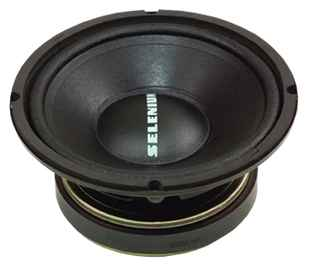open a bigger photo

8W4P       order no. sel-28011062- not available
19 cm bass-midrange driver with high quality cone.
• power handling (continuous/programme) = 300/600 W
• frequency range = 100-6500 Hz
• resonance frequency fs = 107 Hz
• impedance R = 8 Ohm
• sound pressure level SPL = 96 dB (2,83V; 1m)
• DC resistance Re = 5,2 Ohm
• force factor BL = 9,8 N/A
• voice coil inductance L = 1,186 mH
• effective piston radiating area Sd = 250 cm2
• effective mechanical mass incl. air load mms = 20,2 g
• equivalent volume of compliance Vas = 9,2 l
• total Q factor Qts = 0,7 (Qms=13,2, Qes=0,74)
• voice coil diameter = 46 mm
• mounting diameter d = 183 mm
• overall diameter d = 205 mm
• mounting depth (not countersunk) t = 93 mm
• mass m = 3,6 kg
PDF data sheetopen a bigger photo

12PW3-SLF       order no. sel-28011031- not available
29 cm bass-midrange driver with high quality cone.
• power handling (continuous/programme) = 175/400 W
• frequency range = 55-5000 Hz
• resonance frequency fs = 50 Hz
• impedance R = 8 Ohm
• sound pressure level SPL = 97 dB (2,83V; 1m)
• DC resistance Re = 6,4 Ohm
• force factor BL = 9,9 N/A
• voice coil inductance L = 1,611 mH
• effective piston radiating area Sd = 510 cm2
• effective mechanical mass incl. air load mms = 36,9 g
• equivalent volume of compliance Vas = 97 l
• total Q factor Qts = 0,69 (Qms=7,95, Qes=0,75)
• voice coil diameter = 46 mm
• mounting diameter d = 279 mm
• overall diameter d = 308 mm
• mounting depth (not countersunk) t = 124 mm
• mass m = 3,94 kg
PDF data sheet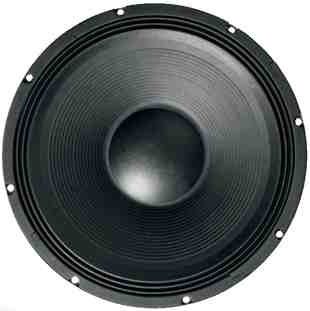open a bigger photo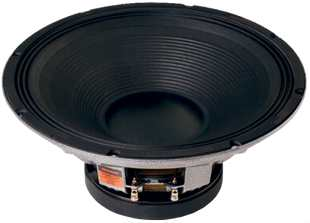open a bigger photo

recommended cabinet 1:
closed cabinet with 41 L volume
from 73/46 Hz (-3dB/-8dB)

recommended cabinet 2:
156 L volume bass reflex cabinet
with 2x HP100 reflex tube, 10 cm long.
from 36/29 Hz (-3dB/-8dB).

15SWS1100       order no. sel-28026130- not available
37 cm bass driver with high quality cone. The basket is made of die-cast.
• power handling (continuous/programme) = 2200/4400 W
• frequency range = 35-3000 Hz
• resonance frequency fs = 37 Hz
• impedance R = 8 Ohm
• sound pressure level SPL = 95 dB (2,83V; 1m)
• DC resistance Re = 5,6 Ohm
• force factor BL = 19,46 N/A
• voice coil inductance L = 7 mH
• effective piston radiating area Sd = 814 cm2
• effective mechanical mass incl. air load mms = 120,1 g
• equivalent volume of compliance Vas = 140 l
• total Q factor Qts = 0,41 (Qms=13,2, Qes=0,42)
• voice coil diameter = 100 mm
• mounting diameter d = 349 mm
• overall diameter d = 389 mm
• mounting depth (not countersunk) t = 195 mm
• mass m = 13,92 kg
PDF data sheetopen a bigger photo

recommended cabinet 1:
closed cabinet with 67 L volume
from 63/40 Hz (-3dB/-8dB)

recommended cabinet 2:
255 L volume bass reflex cabinet
with 4x HP100 reflex tube, 24 cm long.
from 31/25 Hz (-3dB/-8dB).

18SWS800       order no. sel-28026131- not available
44 cm bass driver with high quality cone. The basket is made of die-cast.
• power handling (continuous/programme) = 1600/3200 W
• frequency range = 30-1500 Hz
• resonance frequency fs = 32 Hz
• impedance R = 8 Ohm
• sound pressure level SPL = 96 dB (2,83V; 1m)
• DC resistance Re = 5,8 Ohm
• force factor BL = 24,5 N/A
• voice coil inductance L = 7,983 mH
• effective piston radiating area Sd = 1194 cm2
• effective mechanical mass incl. air load mms = 219 g
• equivalent volume of compliance Vas = 229 l
• total Q factor Qts = 0,41 (Qms=13,72, Qes=0,42)
• voice coil diameter = 100 mm
• mounting diameter d = 420 mm
• overall diameter d = 457 mm
• mounting depth (not countersunk) t = 215 mm
• mass m = 10,5 kg
PDF data sheet

The cabinet volume calculations are executed considering an external resistance of 0.3 Ohm. We are using, if no other data is known or available, the information supplied by the manufacturer.

Please note: the frequency response doesn't say much about the cut-off frequency inside the cabinet! Therefore, we mostly give you 2 examples with dimensioning considerations and an indication, how low the loudspeaker will reproduce.

Home